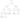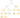# House Robber

## Problem#

There is a robber planning to rob houses along a street. Each house has some amount of money that can be robbed. The robber can rob each house except that adjacent houses cannot be robbed since they have security systems connected and it will automatically contact the police.

Given an integer array nums representing the amount of money of each house, return the maximum amount of money the robber can get without alerting the police.

For example,

```Input: nums = [1,2,3,1]
Output: 4
Explanation: Rob house 1 (money = 1) and then rob house 3 (money = 3).
Total amount you can rob = 1 + 3 = 4.
```
```Input: nums = [2,7,9,3,1]
Output: 12
Explanation: Rob house 1 (money = 2), rob house 3 (money = 9) and rob house 5 (money = 1).
Total amount you can rob = 2 + 9 + 1 = 12.
```

Constraints:

• `1 <= nums.length <= 100`
• `0 <= nums[i] <= 400`

## Solution#

### Brute Force#

Before coming up with dynamic programming solution, let’s define three things:

1. A function or data structure that answers the problem for a given state
`Rob(i)` denotes a function that returns the maximum amount of money that can be robbed until we are at ith house

2. A recurrence relation that defines transition between states

`Math.Max(Rob(i - 1), Rob(i - 2) + nums[i])`

At any house, we have to compare the result between robbery result from previous house or robbery result from previous two house plus the money in current house. This is because we cannot rob from two adjacent houses.

3. Base cases

`Rob(0) = nums[i]` and `Rob(1) = Math.Max(nums, nums)```````public int Rob(int[] nums)
{
return Rob(nums.Length - 1);

int Rob(int i)
{
if (i == 0)
return nums[i];

if (i == 1)
return Math.Max(nums, nums);

return Math.Max(Rob(i - 1), Rob(i - 2) + nums[i]);
}
}
``````

#### Complexity Analysis#

Suppose `N` is the length of `nums`.

• Time Complexity: At each step we generate two subtree and the height of the tree is `N`. The total number of generated nodes is 2N. Since, we traverse all the nodes, then the complexity will be O(2N).
• Space Complexity: The complexity will be the same with O(recursion depth), thus it will be O(N).

### Top-down Dynamic Programming#

If we notice recursion tree below, there are some repeated states such as `Rob(2)`. We can improve the performance by caching the result.

In this case, we use array to cache the result (memoization) and the size is 100 because this is problem’s constraint. Alternatively, we can use `map` like `Dictionary` in C# or `HashMap` in Java that doesn’t require specifying capacity.``````public int Rob(int[] nums)
{
int[] dp = new int;
Array.Fill(dp, -1);

return Rob(nums.Length - 1);

int Rob(int i)
{
if (i == 0)
return nums[i];

if (i == 1)
return Math.Max(nums, nums);

if (dp[i] > -1)
return dp[i];

int ans = Math.Max(Rob(i - 1), Rob(i - 2) + nums[i]);
dp[i] = ans;

return ans;
}
}
``````

#### Complexity Analysis#

Suppose `N` is the length of `nums`.

• Time Complexity: Since we cache each `Rob(i)`, then we will perform at most N recursive calls, thus the complexity is O(N).
• Space Complexity: The complexity will be the same with O(recursion depth), thus it will be O(N).

### Bottom-up Dynamic Programming#

We can avoid recursion stack overhead by using `for-loop`.

``````public int Rob(int[] nums)
{
if (nums.Length == 1)
return nums;

int[] dp = new int[nums.Length];

dp = nums;
dp = Math.Max(nums, nums);

for (int i = 2; i < dp.Length; i++)
{
dp[i] = Math.Max(dp[i - 1], dp[i - 2] + nums[i]);
}

return dp[nums.Length - 1];
}
``````

#### Complexity Analysis#

Suppose `N` is the length of `nums`.

• Time Complexity: Since we iterate linearly up to `N`, then the complexity is O(N).
• Space Complexity: O(N) since we allocate memory as many as `N` to array `dp` for caching.